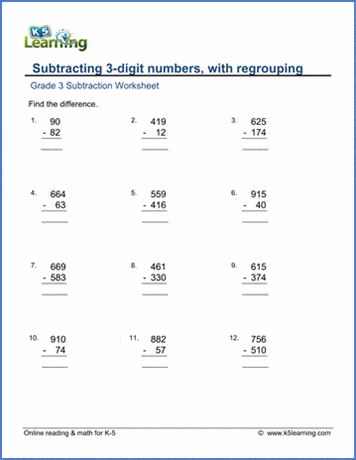# Three-digit Subtraction with Regrouping

## What is regrouping?

When you arrange something into a new group or groups, you regroup. Applied to subtraction, this means regrouping numbers into new groups.

We often use columns when faced with subtraction problems with regrouping. It’s easier to apply the regrouping when the ones are stacked on top of each other, the tens are stacked above each other, and the hundreds are stacked above each other.

We now think of the ones as one group, the tens as one group, the hundreds as one group, etc.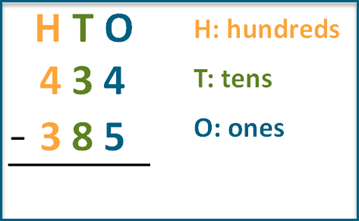## When do you use regrouping?

Regrouping is used when the sum of the values in one place value column (or group as we referred to above) is more than nine.

For example, let’s use the above example: 434 - 385. In this case, you need to regroup. Let’s take this step by step. We start with the ones column. We need to borrow 10 from the tens column as 4 is less than 5. By borrowing 10, we now have 14 – 5, which makes 9.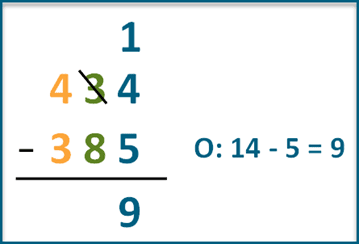Now, turn your attention to the tens. We have 20 – 80, so we’ll again need to borrow from the hundreds. That makes it 120 – 80 = 40.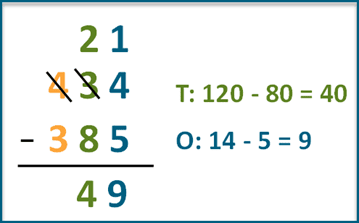Finally, we subtract the hundreds: 300 – 300 = 0.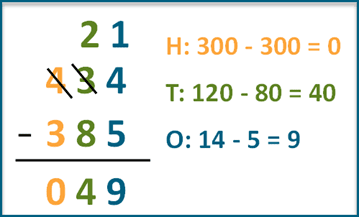## Subtraction with two regroupings video

We’ve created a 60 second video to demonstrate visually how to subtract three-digit numbers with regrouping.

## Grade 2 worksheets to practice 3-digit numbers with borrowing

We’ve created a set of subtraction of 3-digit numbers with regrouping.

https://www.k5learning.com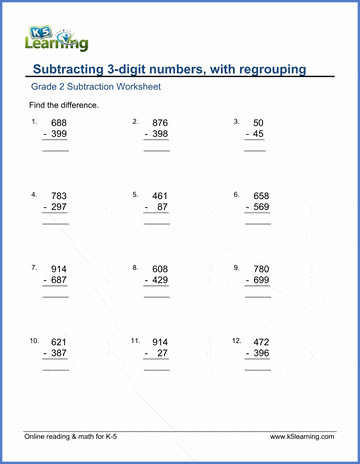## Practice 3-digit subtraction with regrouping at grade 3 level

A set of harder worksheets for 3-digit subtraction with regrouping is available in our grade 3 math section.